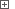# Expanding and Collapsing Rows in Syncfusion GridDataBoundGridThe GridDataBoundGrid control may display data of multiple nested relations. In this case, the grid displays the expand buttons (“+” or “-”) on the left of the data rows that have child data. Expanding a row of this kind provides access to the row’s nested table data.

You can expand and collapse grid rows in two ways:

• By simulating a mouse click over the row’s expand button.

• By using internal methods of the GridDataBoundGrid control.

The click approach is quite complicated. It requires you to know the coordinates of the “+”/“-” button within the grid, which is not an easy task. We will not show this approach here. Below, we will explain how to expand and collapse rows in GridDataBoundGrid control using its internal methods. This approach is much easier and stable than simulating expand button clicks.

The following table lists the GridDataBoundGrid control methods that you can use to expand and collapse its rows:

Method Description
`ExpandAtRowIndex(RowIndex)` Expands the grid row specified by its zero-based absolute index. (For more information on identifying rows in the GridDataBoundGrid control, see Accessing Rows, Columns and Cells in Syncfusion GridDataBoundGrid.)
`CollapseAtRowIndex(RowIndex)` Collapses the grid row specified by its zero-based absolute index.
`IsExpandedAtRowIndex(RowIndex)` Returns True if the specified grid row is expanded, and False if it is collapsed.

The examples below demonstrate how to expand and collapse GridDataBoundGrid rows from scripts.

###### Example 1View description

JavaScript, JScript

function Main ()
{
var p, Grid;

// Obtain the application process and the grid object
p = Sys.Process("ExpandGrid");
p.WinFormsObject("Form1").Maximize();

// Expand and collapse several rows
ExpandRow (Grid, 11);
aqUtils.Delay (500);
CollapseRow (Grid, 11);
aqUtils.Delay (500);
ExpandRow (Grid, 4);
aqUtils.Delay (500);
ExpandRow (Grid, 7);
}

function ExpandRow (Grid, RowIndex)
{
if (Grid.IsExpandedAtRowIndex(RowIndex))
Log.Message ("Row " + RowIndex + " is already expanded.");
else
Grid.ExpandAtRowIndex (RowIndex);
}

function CollapseRow (Grid, RowIndex)
{
if (Grid.IsExpandedAtRowIndex(RowIndex))
Grid.CollapseAtRowIndex (RowIndex);
else
Log.Message ("Row " + RowIndex + " is already collapsed.");
}

Python

``````def Main ():

# Obtain the application process and the grid object
p = Sys.Process("ExpandGrid")
p.WinFormsObject("Form1").Maximize()

# Expand and collapse several rows
ExpandRow (Grid, 11)
aqUtils.Delay (500)
CollapseRow (Grid, 11)
aqUtils.Delay (500)
ExpandRow (Grid, 4)
aqUtils.Delay (500)
ExpandRow (Grid, 7)

def ExpandRow (Grid, RowIndex):
if (Grid.IsExpandedAtRowIndex(RowIndex)):
Log.Message ("Row " + RowIndex + " is already expanded.")
else:
Grid.ExpandAtRowIndex (RowIndex)

def CollapseRow (Grid, RowIndex):
if (Grid.IsExpandedAtRowIndex(RowIndex)):
Grid.CollapseAtRowIndex (RowIndex)
else:
Log.Message ("Row " + RowIndex + " is already collapsed.")``````

VBScript

Sub Main
Dim p, Grid

' Obtain the application process and the grid object
Set p = Sys.Process("ExpandGrid")
p.WinFormsObject("Form1").Maximize

' Expand and collapse several rows
Call ExpandRow (Grid, 11)
Call aqUtils.Delay (500)
Call CollapseRow (Grid, 11)
Call aqUtils.Delay (500)
Call ExpandRow (Grid, 4)
Call aqUtils.Delay (500)
Call ExpandRow (Grid, 7)
End Sub

Sub ExpandRow (Grid, RowIndex)
If Grid.IsExpandedAtRowIndex(RowIndex) Then
Call Log.Message ("Row " & RowIndex & " is already expanded.")
Else
Call Grid.ExpandAtRowIndex (RowIndex)
End If
End Sub

Sub CollapseRow (Grid, RowIndex)
If Grid.IsExpandedAtRowIndex(RowIndex) Then
Call Grid.CollapseAtRowIndex (RowIndex)
Else
Call Log.Message ("Row " & RowIndex & " is already collapsed.")
End If
End Sub

DelphiScript

procedure ExpandRow (Grid, RowIndex); forward;
procedure CollapseRow (Grid, RowIndex); forward;

procedure Main;
var p, Grid : OleVariant;
begin
// Obtain the application process and the grid object
p := Sys.Process('ExpandGrid');
p.WinFormsObject('Form1').Maximize;

// Expand and collapse several rows
ExpandRow (Grid, 11);
aqUtils.Delay (500);
CollapseRow (Grid, 11);
aqUtils.Delay (500);
ExpandRow (Grid, 4);
aqUtils.Delay (500);
ExpandRow (Grid, 7);
end;

procedure ExpandRow (Grid, RowIndex);
begin
if Grid.IsExpandedAtRowIndex(RowIndex) then
Log.Message ('Row ' + aqConvert.VarToStr(RowIndex) + ' is already expanded.')
else
Grid.ExpandAtRowIndex (RowIndex);
end;

procedure CollapseRow (Grid, RowIndex);
begin
if Grid.IsExpandedAtRowIndex(RowIndex) then
Grid.CollapseAtRowIndex (RowIndex)
else
Log.Message ('Row ' + aqConvert.VarToStr(RowIndex) + ' is already collapsed.');
end;

C++Script, C#Script

function Main ()
{
var p, Grid;

// Obtain the application process and the grid object
p = Sys["Process"]("ExpandGrid");
p["WinFormsObject"]("Form1")["Maximize"]();

// Expand and collapse several rows
ExpandRow (Grid, 11);
aqUtils["Delay"] (500);
CollapseRow (Grid, 11);
aqUtils["Delay"] (500);
ExpandRow (Grid, 4);
aqUtils["Delay"] (500);
ExpandRow (Grid, 7);
}

function ExpandRow (Grid, RowIndex)
{
if (Grid["IsExpandedAtRowIndex"](RowIndex))
Log["Message"]("Row " + RowIndex + " is already expanded.");
else
Grid["ExpandAtRowIndex"](RowIndex);
}

function CollapseRow (Grid, RowIndex)
{
if (Grid["IsExpandedAtRowIndex"](RowIndex))
Grid["CollapseAtRowIndex"](RowIndex);
else
Log["Message"]("Row " + RowIndex + " is already collapsed.");
}

###### Example 2

To expand or collapse all grid rows at once, you can use the `ExpandAll` and `CollapseAll` methods:

JavaScript, JScript

function Main ()
{
var Grid;
// Obtain the grid object
...

// Expand all rows
Grid.ExpandAll();
...

// Collapse all rows
Grid.CollapseAll();
...
}

Python

``````def Main ():
# Obtain the grid object
...

# Expand all rows
Grid.ExpandAll()
...

# Collapse all rows
Grid.CollapseAll()
...``````

VBScript

Sub Main
Dim Grid
' Obtain the grid object
...

' Expand all rows
Grid.ExpandAll
...

' Collapse all rows
Grid.CollapseAll
...
End Sub

DelphiScript

procedure Main;
var Grid : OleVariant;
begin
// Obtain the grid object
...

// Expand all rows
Grid.ExpandAll;
...

// Collapse all rows
Grid.CollapseAll;
...
end;

C++Script, C#Script

function Main ()
{
var Grid;
// Obtain the grid object
...

// Expand all rows
Grid["ExpandAll"]();
...

// Collapse all rows
Grid["CollapseAll"]();
...
}